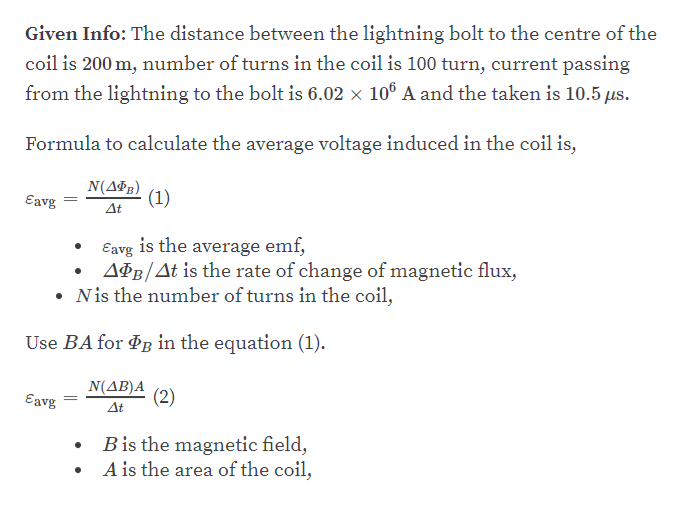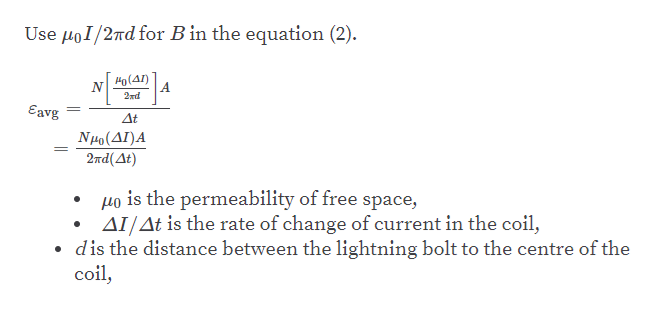Question
8 views

The bolt of lightning depicted in Figure P20.61 passes 200. m
from a 100-turn coil oriented as shown. If the current in the

lightning bolt falls from 6.02 x 106 A to zero in 10.5 µs, what
is the average voltage induced in the coil? Assume the distance
to the center of the coil determines the average magnetic
field at the coil’s position. Treat the lightning bolt as a
long, vertical wire.

check_circle

Step 1help_outlineImage TranscriptioncloseGiven Info: The distance between the lightning bolt to the centre of the coil is 200 m, number of turns in the coil is 100 turn, current passing from the lightning to the bolt is 6.02 x 10° A and the taken is 10.5 us. Formula to calculate the average voltage induced in the coil is, N(Δ) (1) At Eavg Eavg is the average emf, AðB/At is the rate of change of magnetic flux, Nis the number of turns in the coil, Use BA for Pg in the equation (1). N(AB)A (2) Eavg At Bis the magnetic field, A is the area of the coil, fullscreen
Step 2help_outlineImage TranscriptioncloseUse µoI/2nd for B in the equation (2). Ho(41) A. 2жd Eavg At Nµo(AI)A 2nd( Де) µo is the permeability of free space, Orl AI/At is the rate of change of current in the coil, • dis the distance between the lightning bolt to the centre of the coil, fullscreen

### Want to see the full answer?

See Solution

#### Want to see this answer and more?

Solutions are written by subject experts who are available 24/7. Questions are typically answered within 1 hour.*

See Solution
*Response times may vary by subject and question.
Tagged in

### Other